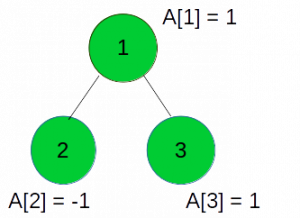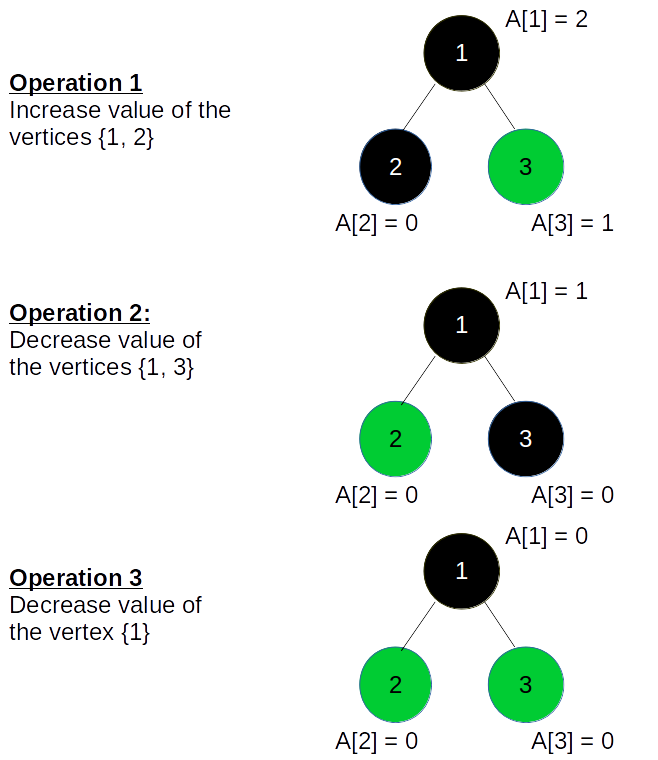# Minimum Operations to make value of all vertices of the tree Zero

Given a tree, where each vertex V has a value A[V] stored in it. The task is to find the minimum number of operations required to make the values stored in all vertices of the tree equal to zero.

Each Operation consists of following 2 steps:

1. Select a Subtree such that the subtree include vertex 1.
2. Increase/Decrease the value of all vertices of the subtree by 1.

Consider the following tree:Note: The number in the vertex denotes the vertex number and A[V] denotes the value of the vertex as explained above.

For the following Tree we perform the following 3 operations to make the values all the vertices
equal to zero:Note: The vertices in black represent the subtree selected.

We can solve this problem using Dynamic Programming.

Let dp[i] denote the number of operations where any subtree rooted at i is selected and the value of all the vertices is increased by 1.
Similarly, dp[i] denotes the number of operations where any subtree rooted at i is selected and the value of all the vertices is decreased by 1.

For all the leaves we can easily compute dp[i] and dp[i] if say a leaf node V is such that A[V] = 0 for some leaf node U, i.e dp[i] = A[V] and dp[i] = 0

Now if we are in some non leaf node say v, we look at all of its children, if say increase operation is applied Xi times for a child i of V then we need to apply max(Xi for all children i of node v), increase operations for any subtree rooted at v. Similarly we do the same for Decrease operations for the node V.

The answer is the sum of the increase and decrease operations for node 1 since the operations are applied only on subtrees having node 1.

Below is the implementation of the above approach:

## C++

 `// CPP program to find the Minimum Operations ` `// to modify values of all tree vertices to zero ` `#include ` ` `  `using` `namespace` `std; ` ` `  `// A utility function to add an edge in an ` `// undirected graph ` `void` `addEdge(vector<``int``> adj[], ``int` `u, ``int` `v) ` `{ ` `    ``adj[u].push_back(v); ` `    ``adj[v].push_back(u); ` `} ` ` `  `// A utility function to print the adjacency list ` `// representation of graph ` `void` `printGraph(vector<``int``> adj[], ``int` `V) ` `{ ` `    ``for` `(``int` `v = 0; v < V; ++v) { ` `        ``cout << ``"\n Adjacency list of vertex "` `             ``<< v << ``"\n head "``; ` `        ``for` `(``auto` `x : adj[v]) ` `            ``cout << ``"-> "` `<< x; ` `        ``printf``(``"\n"``); ` `    ``} ` `} ` ` `  `// Utility Function for findMinOperation() ` `void` `findMinOperationUtil(``int` `dp[], vector<``int``> adj[], ` `                          ``int` `A[], ``int` `src, ``int` `parent) ` `{ ` `    ``// Base Case for current node ` `    ``dp[src] = dp[src] = 0; ` ` `  `    ``// iterate over the adjacency list for src ` `    ``for` `(``auto` `V : adj[src]) { ` `        ``if` `(V == parent) ` `            ``continue``; ` ` `  `        ``// calculate DP table for each child V ` `        ``findMinOperationUtil(dp, adj, A, V, src); ` ` `  `        ``// Number of Increase Type operations for node src ` `        ``// is equal to maximum of number of increase operations ` `        ``// required by each of its child ` `        ``dp[src] = max(dp[src], dp[V]); ` ` `  `        ``// Number of Decrease Type operations for node src ` `        ``// is equal to maximum of number of decrease operations ` `        ``// required by each of its child ` `        ``dp[src] = max(dp[src], dp[V]); ` `    ``} ` ` `  `    ``// After performing operations for subtree rooted at src ` `    ``// A[src] changes by the net difference of increase and ` `    ``// decrease type operations ` `    ``A[src - 1] += dp[src] - dp[src]; ` ` `  `    ``// for negative value of node src ` `    ``if` `(A[src - 1] > 0) { ` `        ``dp[src] += A[src - 1]; ` `    ``} ` `    ``else` `{ ` `        ``dp[src] += ``abs``(A[src - 1]); ` `    ``} ` `} ` ` `  `// Returns the minimum operations required to make ` `// value of all vertices equal to zero, uses ` `// findMinOperationUtil() ` `int` `findMinOperation(vector<``int``> adj[], ``int` `A[], ``int` `V) ` `{ ` ` `  `    ``// Initialise DP table ` `    ``int` `dp[V + 1]; ` `    ``memset``(dp, 0, ``sizeof` `dp); ` ` `  `    ``// find dp and dp ` `    ``findMinOperationUtil(dp, adj, A, 1, 0); ` ` `  `    ``int` `minOperations = dp + dp; ` `    ``return` `minOperations; ` `} ` ` `  `// Driver code ` `int` `main() ` `{ ` `    ``int` `V = 5; ` ` `  `    ``// Build the Graph/Tree ` `    ``vector<``int``> adj[V + 1]; ` `    ``addEdge(adj, 1, 2); ` `    ``addEdge(adj, 1, 3); ` ` `  `    ``int` `A[] = { 1, -1, 1 }; ` `    ``int` `minOperations = findMinOperation(adj, A, V); ` `    ``cout << minOperations; ` ` `  `    ``return` `0; ` `} `

## Python3

 `# Python3 program to find the Minimum Operations  ` `# to modify values of all tree vertices to zero  ` ` `  `# A utility function to add an  ` `# edge in an undirected graph  ` `def` `addEdge(adj, u, v):  ` ` `  `    ``adj[u].append(v)  ` `    ``adj[v].append(u)  ` ` `  `# A utility function to print the adjacency  ` `# list representation of graph  ` `def` `printGraph(adj, V):  ` ` `  `    ``for` `v ``in` `range``(``0``, V):  ` `        ``print``(``"Adjacency list of vertex"``, v) ` `        ``print``(``"head"``, end ``=` `" "``) ` `         `  `        ``for` `x ``in` `adj[v]:  ` `            ``print``(``"->"``, x, end ``=` `"")  ` `        ``print``()  ` ` `  `# Utility Function for findMinOperation()  ` `def` `findMinOperationUtil(dp, adj, A, src, parent):  ` ` `  `    ``# Base Case for current node  ` `    ``dp[src][``0``] ``=` `dp[src][``1``] ``=` `0` ` `  `    ``# Iterate over the adjacency list for src  ` `    ``for` `V ``in` `adj[src]:  ` `        ``if` `V ``=``=` `parent:  ` `            ``continue` ` `  `        ``# calculate DP table for each child V  ` `        ``findMinOperationUtil(dp, adj, A, V, src)  ` ` `  `        ``# Number of Increase Type operations for node src  ` `        ``# is equal to maximum of number of increase operations  ` `        ``# required by each of its child  ` `        ``dp[src][``0``] ``=` `max``(dp[src][``0``], dp[V][``0``])  ` ` `  `        ``# Number of Decrease Type operations for node  ` `        ``# src is equal to maximum of number of decrease  ` `        ``# operations required by each of its child  ` `        ``dp[src][``1``] ``=` `max``(dp[src][``1``], dp[V][``1``])  ` `     `  `    ``# After performing operations for subtree rooted  ` `    ``# at src A[src] changes by the net difference of  ` `    ``# increase and decrease type operations  ` `    ``A[src ``-` `1``] ``+``=` `dp[src][``0``] ``-` `dp[src][``1``]  ` ` `  `    ``# for negative value of node src  ` `    ``if` `A[src ``-` `1``] > ``0``: ` `        ``dp[src][``1``] ``+``=` `A[src ``-` `1``]  ` `     `  `    ``else``: ` `        ``dp[src][``0``] ``+``=` `abs``(A[src ``-` `1``])  ` ` `  `# Returns the minimum operations required to  ` `# make value of all vertices equal to zero,  ` `# uses findMinOperationUtil()  ` `def` `findMinOperation(adj, A, V):  ` ` `  `    ``# Initialise DP table  ` `    ``dp ``=` `[[``0``, ``0``] ``for` `i ``in` `range``(V ``+` `1``)]  ` ` `  `    ``# find dp and dp  ` `    ``findMinOperationUtil(dp, adj, A, ``1``, ``0``)  ` ` `  `    ``minOperations ``=` `dp[``1``][``0``] ``+` `dp[``1``][``1``]  ` `    ``return` `minOperations  ` ` `  `# Driver code  ` `if` `__name__ ``=``=` `"__main__"``:  ` ` `  `    ``V ``=` `5` ` `  `    ``# Build the Graph/Tree  ` `    ``adj ``=` `[[] ``for` `i ``in` `range``(V ``+` `1``)] ` `    ``addEdge(adj, ``1``, ``2``)  ` `    ``addEdge(adj, ``1``, ``3``)  ` ` `  `    ``A ``=` `[``1``, ``-``1``, ``1``] ` `    ``minOperations ``=` `findMinOperation(adj, A, V)  ` `    ``print``(minOperations)  ` ` `  `# This code is contributed by Rituraj Jain `

Output:

```3
```

Time Complexity : O(V), where V is the number of nodes in the tree.
Auxiliary Space : O(V), where V is the number of nodes in the tree.

My Personal Notes arrow_drop_upCheck out this Author's contributed articles.

If you like GeeksforGeeks and would like to contribute, you can also write an article using contribute.geeksforgeeks.org or mail your article to contribute@geeksforgeeks.org. See your article appearing on the GeeksforGeeks main page and help other Geeks.

Please Improve this article if you find anything incorrect by clicking on the "Improve Article" button below.

Improved By : rituraj_jain